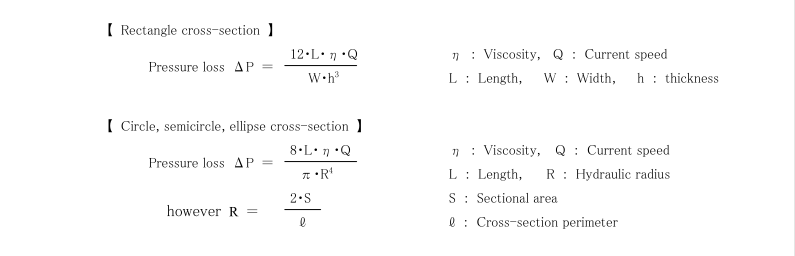## Pressure loss will occur on the sprue, runner, and gate part so be careful.

Time: 2020-08-10
Summary: Practically, resin is a non Newtonian fluid with viscoelasticity, so viscosity will decrease if the shearing force get bigger and cross-section thickness and diameter get smaller. So the actual effect of pressure loss might be bit smaller than the formula above.Pressure loss will occur on the sprue, runner, and gate part so be careful. Presuming the resin is Newtonian fluid, the amount of pressure loss will be shown as the following, and from that, we know it depends largely on cross-section thickness and diameter.
Practically, resin is a non Newtonian fluid with viscoelasticity, so viscosity will decrease if the shearing force get bigger and cross-section thickness and diameter get smaller. So the actual effect of pressure loss might be bit smaller than the formula above.